﻿﻿﻿ Flow coefficient kv, cv ﻿ ﻿ ﻿

# Flow coefficient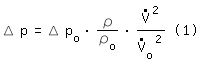Δ p,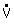, ρ : Pressure loss, Volume flow and Density of component´s point of operation Δ po,o, ρo : Pressure loss, Volume flow and Density of component´s reference point of operation

The valve manufacturer Mason-Neilan (MA, USA) introduced in the 1940s the flow coefficient cv . In the 1950s cv was introduced as kv -value   (kvs-value for rated travel) in the metric system [Früh 1957]. The flow coefficients cv and kv describe a valve´s reference point of operation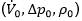. The reference fluid is water (ρo = density of water at 15,6 ºC) [VDI/VDE 2173-2007 page 8].

 kv :o = kv [m³/h] @   Δpo = 1 [bar] cv,us :o = cv,us [USgal/min] @   Δpo = 1 [psi] ≡o[m³/h] = 0.2271 cv,us [USgal/min] @   Δpo = 0.0689 [bar] cv,uk :o = cv,uk [UKgal/min] @   Δpo = 1 [psi] ≡o[m³/h] = 0.2728 cv,uk [UKgal/min] @   Δpo = 0.0689 [bar]

Putting equation (1) towe obtain the correlation between kv and cv   (ρ/ρo=1):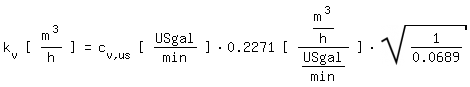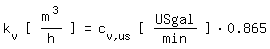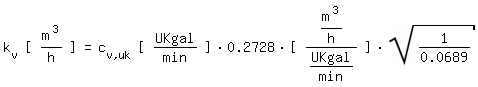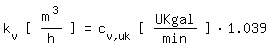Equation (1) is derived from equation (2). Equation (2) is derived from the equation of Darcy-Weisbach :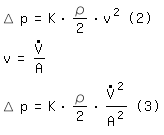K : Dimensionless resistance coefficient (friction factor) of the component. K is assumed to be constant. v : Average speed in the characteristic cross section A : Characteristic cross section d : Inner diameter of the characteristic cross section.For valves as a general rule the characteristic diameter equals the nominal diameter DN.

The resistance coefficient K is assumed to be constant. This assumption is correct for completely turbulent flow. In many other cases the inaccuracy is acceptable.
Putting equation (3) to K and with A = π/4 d2 the correlation between resistance coefficient K and flow coefficient kv becomes equation (5):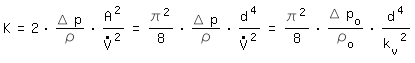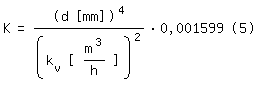By lowering the pressure behind a valve the volume flow can´t rise without limits. Pressures below the vapour pressure lead to cavitation. The noise level rises and at maximum cavitation we face choked flow. According to [VDI/VDE 2173-2007 page 10] we can note for control valves: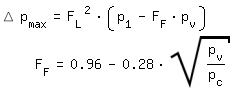p1: Absolute pressure at the inlet
pv: Vapour pressure
pc: Critical pressure (Water: pc=221,2 bar)

 Ball valve : FL= 0.5-0.7 Butterfly valve (aperture angle 60-70º) : FL= 0.55-0.75 Low-noise control valve : FL= 0.88-0.98

More detailed information (including not turbulent flow) can be found in [DIN EN 60534 2012].

 Literature [DIN EN 60534 2012] DIN EN 60534-2-1: Stellventile für die Prozessregelung, Beuth-Verlag Berlin, Januar 2012 [Früh 1957] K.F.Früh: Berechnung des Durchflusses in Regelventilen mit Hilfe des kv-Koeffizienten, Regelungstechnik Heft 9; 5. Jahrgang 1957 [VDI/VDE 2173-2007] VDI/VDE 2173: Stömungstechnische Kenngrössen von Stellventilen und deren Bestimmung, Beuth-Verlag Berlin, September 2007

﻿